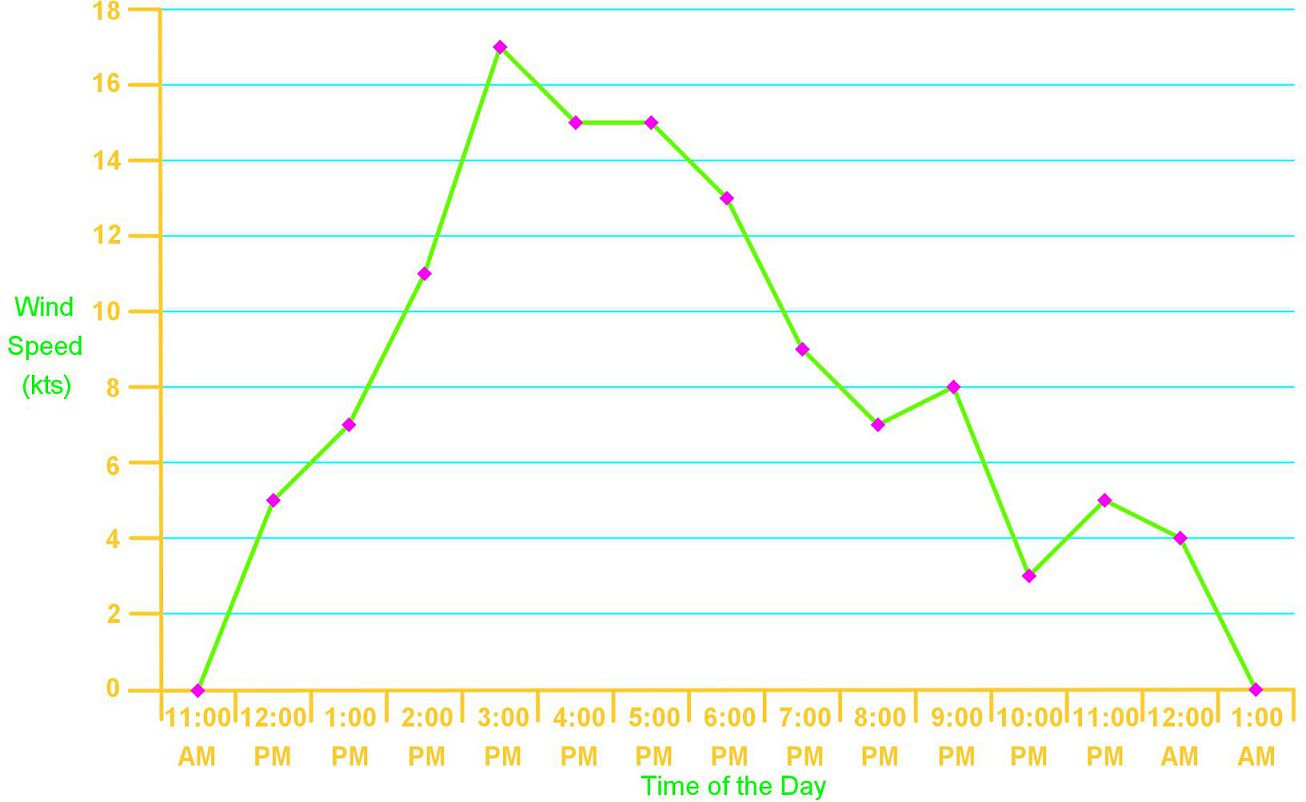# Frequency polygons

### Frequency polygons

#### Lessons

Frequency Polygons are graphs that are constructed out of a frequency table. To create a Frequency Polygon just follow these steps:
1) Create a histogram
2) Plot the midpoints for each bar on the histogram
3) Plot a point with a value of zero one bar below the smallest class. Also plot a point with a value of zero one bar above the largest class
4) Connect all the data point with a straight line
• Introduction
What are frequency polygons?

• 1.
Constructing a Frequency Polygon from a Frequency Distribution
The following frequency table shows the amount of time it took 45 ski racers to complete a ski course:
 Lower Limit: Upper Limit: Frequency: Cumulative Frequency: 80 seconds 86 seconds 3 3 86 seconds 92 seconds 5 8 92 seconds 98 seconds 12 20 98 seconds 104 seconds 8 28 104 seconds 110 seconds 16 34 110 seconds 116 seconds 11 45
a)
Using the frequency distribution table construct a frequency polygon

b)
Using the frequency distribution table construct a cumulative frequency polygon

• 2.
Interpreting a Frequency Polygon
The frequency polygon below shows the wind speed during a particular day in San Francisco Bay:a)
What was the highest wind speed recording during this day? What was the lowest wind speed recorded?

b)
During how many hours in this day was the wind speed recorded?

c)
How many hours of the day had more than 10 knots of wind speed?

d)
How many hours of the day had between 2-6 knots of wind speed?